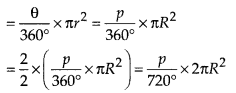# MP Board Class 10th Maths Solutions Chapter 12 Areas Related to Circles Ex 12.2

In this article, we will share MP Board Class 10th Maths Book Solutions Chapter 12 Areas Related to Circles Ex 12.2 Pdf, These solutions are solved subject experts from the latest edition books.

## MP Board Class 10th Maths Solutions Chapter 12 Areas Related to Circles Ex 12.2

Unless stated otherwise, use π = $$\frac{22}{7}$$.

Question 1.
Find the area of a sector of a circle with radius 6 cm if angle of the sector is 60°.
Solution:
Here, r = 6 cm and θ = 60°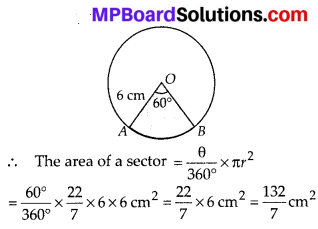Question 2.
Find the area of a quadrant of a circle whose circumference is 22 cm.
Solution:
Let radius of the circle be r.
∴ 2πr = 22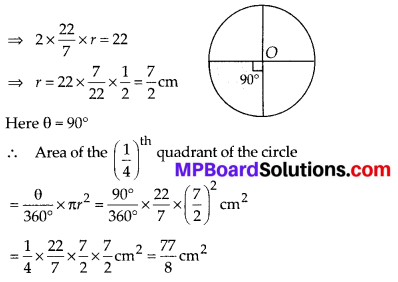Question 3.
The length of the minute hand of a clock is 14 cm. Find the area swept by the minute hand in 5 minutes.
Solution:
Length of minute hand = radius of the circle (r) = 14 cm
∵ Angle swept by the minute hand in 60 minutes = 360°
∴ Angle swept by the minute hand in 360°
5 minutes = $$\frac{360^{\circ}}{60}$$ × 5 = 30°
Now, area of the sector with r = 14 cm and θ = 30°
= $$\frac{\theta}{360^{\circ}}$$ × πr2 = $$\frac{30^{\circ}}{360^{\circ}} \times \frac{22}{7}$$ × 14 × 14 cm2
= $$\frac{11 \times 14}{3} \mathrm{cm}^{2}=\frac{154}{3} \mathrm{cm}^{2}$$
Thus, the required area swept by the minute hand in 5 minutes = $$\frac{154}{3}$$ cm2Question 4.
A chord of a circle of radius 10 cm subtends a right angle at the centre. Find the area of the corresponding: (i) minor segment (ii) major segment.(Use π = 3.14)
Solution:
Length of the radius (r) = 10 cm
Sector angle (θ) = 90°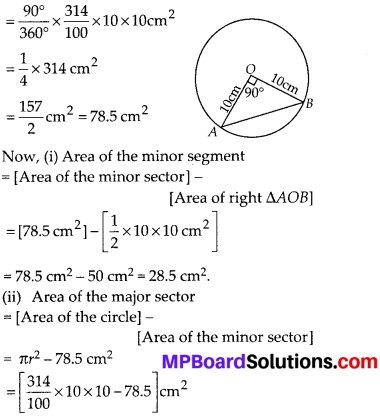= [314 – 78.5] cm2 = 235.5 cm2

Question 5.
In a circle of radius 21 cm, an arc subtends an angle of 60° at the centre. Find:
(i) the length of the arc
(ii) area of the sector formed by the arc
(iii) area of the segment formed by the corresponding chord
Solution:
Here, radius(r) = 21 cm and θ = 60°
(i) Circumference of the circle = 2πr
= 2 × $$\frac{22}{7}$$ × 21 cm = 2 × 22 × 3 cm = 132 cm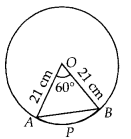∴ Length of the arc APB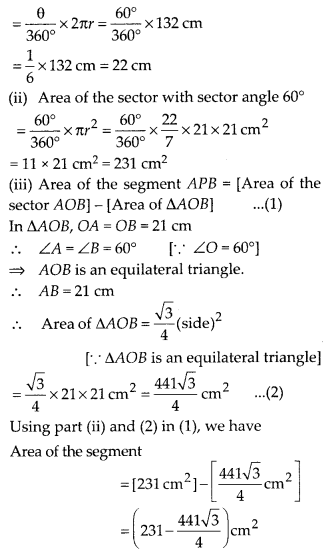Question 6.
A chord of a circle of radius 15 cm subtends an angle of 60° at the centre. Find the areas of the corresponding minor and major segments of the circle. (Use π = 3.14 and $$\sqrt{3}=$$ = 1.73)
Solution:
Here, radius (r) = 15 cm and
Sector angle (θ) = 60°
∴ Area of the sector
$$\frac{\theta}{360^{\circ}}$$ × πr2 = $$\frac{60^{\circ}}{360^{\circ}} \times \frac{314}{100}$$ × 15 × 15 cm2
= $$\frac{11775}{100}$$ cm2 = 117.75 cm2
Since ∠O = 60° and OA = OB = 15 cm
∴ AOB is an equilateral triangle.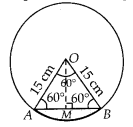⇒ AB = 15 cm and ∠A = 60°
Draw OM ⊥ AB,
In ∆AMO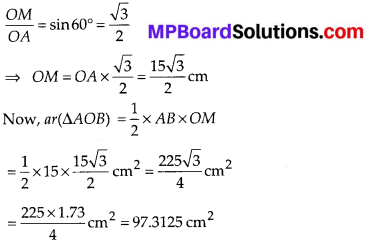Now area of the minor segment = (Area of minor sector) – (ar ∆AOB)
= (117.75 – 97.3125) cm2 = 20.4375 cm2
Area of the major segment = [Area of the circle] – [Area of the minor segment]
= πr2 – 20.4375 cm2
= [$$\frac{314}{100}$$ × 152] – 20.4375 cm2
= (706.5 – 20.4375) cm2 = 686.0625 cm2

Question 7.
A chord of a circle of radius 12 cm subtends an angle of 120° at the centre. Find the area of the corresponding segment of the circle. (Use π = 3.14 and $$\sqrt{3}=$$ = 1.73 )
Solution:
Here θ = 120° and r = 12 cm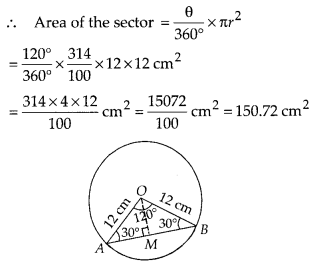Draw OM ⊥ AB
In ∆AOB, ∠O = 120°
By angle sum property,
∠A + ∠B + ∠O = 180°
⇒ ∠A + ∠B = 180° – 120° = 60°
∵ OB = OA = 12 cm
⇒ ∠A = ∠B = 30°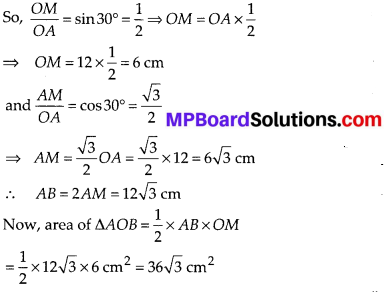= 36 × 1.73 cm2 = 62.28 cm2
∴ Area of the minor segment = [Area of sector] – [Area of ∆AOB]
= [150.72 cm2] – [62.28 cm2] = 88.44 cm2

Question 8.
A horse is tied to a peg at one corner of a square shaped grass field of side 15 m by means of a 5 m long rope (see figure). Find
(i) the area of that part of the field in which the horse can graze.
(ii) the increase in the grazing area if the rope were 10m long instead of 5 m. (Use π = 3.14)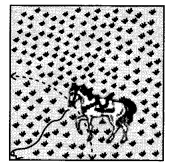Solution:
Here, length of the rope = 5 m
∴ Radius of the circular portion grazed by the horse(r) = 5 m
(i) Area of the circular portion grazed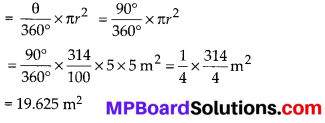(ii) When length of the rope is increased to 10 m, then, r = 10 m
Area of the new circular portion grazed
= $$\frac{\theta}{360^{\circ}}$$ × πr2 = $$\frac{90^{\circ}}{360^{\circ}} \times \frac{314}{100}$$ × (10)2 m2
= $$\frac{1}{4}$$ × 314 m2 = 78.5 m2
∴ Increase in the grazing area = (78.5 – 19.625) m2 = 58.875 m2Question 9.
A brooch is made with silver wire in the form of a circle with diameter 35 mm. The wire is also used in making 5 diameters which divide the circle into 10 equal sectors as shown in the figure. Find:
(i) the total length of the silver wire required.
(ii) the area of each sector of the brooch.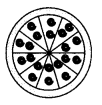Solution:
Diameter of the circle = 35 mm
∴ Radius (r) = $$\frac{35}{2}$$ mm
(i) Circumference = 2πr
= 2 × $$\frac{22}{7} \times \frac{35}{2}$$ mm = 22 × 5 mm = 110 mm
Length of 1 piece of wire used to make diameter to divide the circle into 10 equal sectors = 35 mm
∴ Length of 5 pieces = 5 × 35 mm = 175 mm
∴ Total length of the silver wire = (110 + 175) mm = 285 mm

(ii) Since the circle is divided into 10 equal sectors,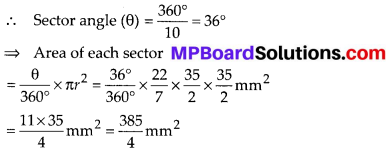Question 10.
An umbrella has 8 ribs which are equally spaced (see figure). Assuming umbrella to be a flat circle of radius 45 cm, find the area between the two consecutive ribs of the umbrella.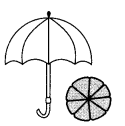Solution:
Here, radius (r) = 45 cm
Since circle is divided into 8 equal parts,
∴ Sector angle corresponding to each part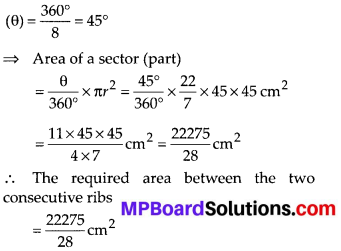Question 11.
A car has two wipers which do not overlap. Each wiper has a blade of length 25 cm sweeping through an angle of 115°. Find the total area cleaned at each sweep of the blades.
Solution:
Here, radius (r) = 25 cm
Sector angle (θ) = 115°
∴ Total area cleaned at each sweep of the blades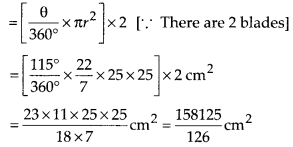Question 12.
To warn ships for underwater rocks, a lighthouse spreads a red coloured light over a sector of angle 80° to a distance of 16.5 km. Find the area of the sea over which the ships are warned. (Use π = 3.14)
Solution:
Here, radius (r) = 16.5 km and sector angle (θ) = 80°
∴ Area of the sea surface over which the ships are warned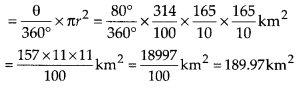Question 13.
A round table cover has six equal designs as shown in the figure. If the radius of the cover is 28 cm, find the cost of making the designs at the rate of ₹ 0.35 per cm2. (Use $$\sqrt{3}=$$ = 1.7)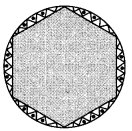Solution:
Here, r = 28 cm
Since, the circle is divided into six equal sectors.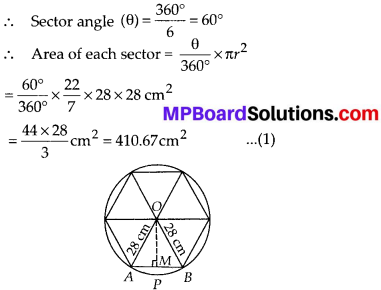Now, area of 1 design = Area of segment APB = Area of sector ABO – Area of ∆AOB ………..(2)
In ∆AOB, ∠AOB = 60°, OA = OB = 28 cm
∴ ∠OAB = 60° and ∠OBA = 60°
⇒ ∆AOB is an equilateral triangle.
⇒ AB = AO = BO ⇒ AB = 28 cm
Draw OM ⊥ AB
∴ In right ∆AOM, we have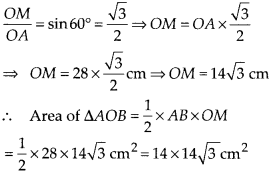= 14 × 14 × 1.7 cm2 = 333.2 cm2 ……………(3)
Now, from (1), (2) and (3), we have
Area of segment APB
= 410.67 cm2 – 333.2 cm2 = 77.47 cm2
⇒ Area of 1 design = 77.47 cm2
∴ Area of the 6 equal designs = 6 × (77.47) cm2 = 464.82 cm2
Hence, the cost of making the design at the rate of ₹ 0.35 per cm2
= ₹ 0.35 × 464.82 = ₹ 162.68.

Question 14.
Tick the correct answer in the following:
Area of a sector of angle p (in degrees) of a circle with radius R is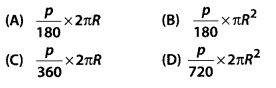Solution: